Next: Formulas for amplitudes (3D Up: Goldin: Method of discontinuities Previous: One-way propagation (PDKO)

# 9: INTEGRAL OPERATORS OF WAVE-FIELD CONTINUATIONS

Kirchoff's type representations of the DKO for the homogeneous medium prompts the general form of an integral KO: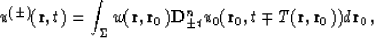(99)

where- negative weight function,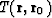- propagation time fromtowith accordance with a given eiconal equation(100)

Without any lack of generality we can put n=0. We propose that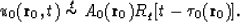then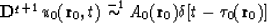and(101)

It is well known that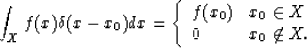It is easy to show that when pointis placed behind the front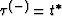(where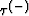is the correspondent solution of equation (100) with condition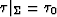), then argument of-function at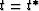is always positive:(see Figure). It means that in this case,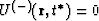. It is also can be shown that whenis between the frontand the surface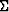, that argument of-function attakes both negative and positive meanings. So in this case,. This proves kinematically equivalence of the operator (99).Next: Formulas for amplitudes (3D Up: Goldin: Method of discontinuities Previous: One-way propagation (PDKO)
Stanford Exploration Project
1/13/1998